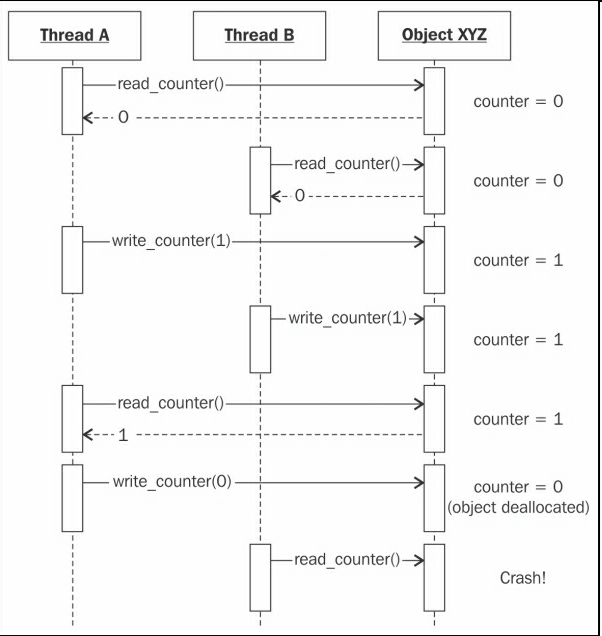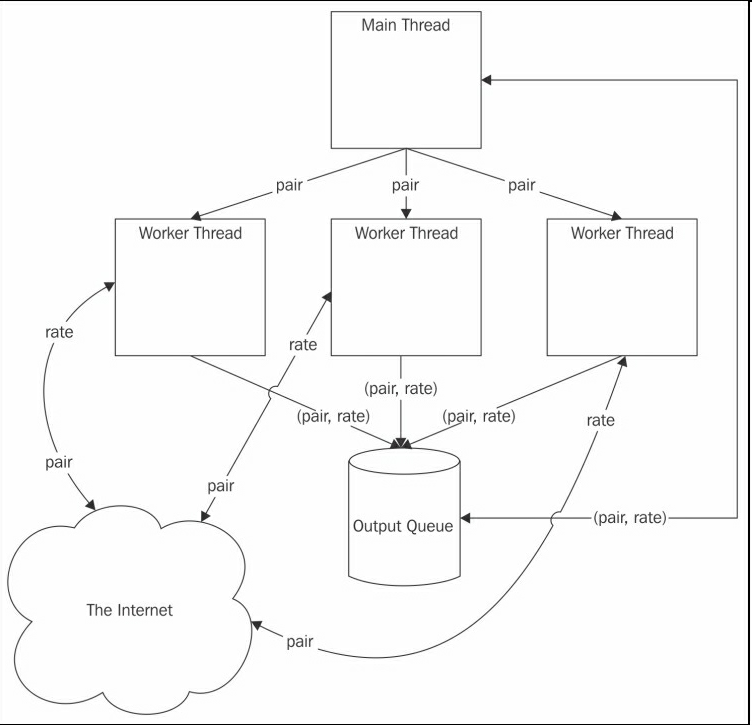#《Python分布式计算》 第3章 Python的并行计算 （Distributed Computing with Python）

0
2
01. 云栖社区>
2. 博客>
3. 正文

## 《Python分布式计算》 第3章 Python的并行计算 （Distributed Computing with Python）

seancheney 2017-10-13 22:56:00 浏览1008

• 多线程
• 多进程
• 多进程队列

# 多线程

Python从1.4版本开始就支持多线程了。它在`threading`模块中还提供了一个高级界面给系统本地（Linux和Mac OS X中的POSIX）线程，本章的例子会使用`threading`

``````from threading import Thread
from queue import Queue
import urllib.request

URL = 'http://finance.yahoo.com/d/quotes.csv?s={}=X&f=p'
def get_rate(pair, outq, url_tmplt=URL):
with urllib.request.urlopen(url_tmplt.format(pair)) as res:
outq.put((pair, float(body.strip())))

if __name__ == '__main__':
import argparse

parser = argparse.ArgumentParser()
args = parser.parse_args()

outputq = Queue()
for pair in args.pairs:
kwargs={'pair': pair,
'outq': outputq})
t.daemon = True
t.start()

for _ in args.pairs:
pair, rate = outputq.get()
print(pair, rate)
outputq.join()
````````````>>> from currency import get_rate
>>> import queue
>>> from time import time
>>> q = queue.Queue()
>>> pairs = ('EURUSD', 'GBPUSD', 'CHFEUR')
>>> t0 = time(); [get_rate(p, q) for p in pairs]; dt = time() - t0
[None, None, None]
>>> dt
1.1785249710083008
>>> [q.get() for p in pairs]
[('EURUSD', 1.1042), ('GBPUSD', 1.5309), ('CHFEUR', 0.9176)]
``````

``````\$ time python3.5 currency.py EURUSD GBPUSD CHFEUR
EURUSD 1.1042
GBPUSD 1.5309
CHFEUR 0.9176
python3.5 currency.py EURUSD GBPUSD CHFEUR  0.08s user 0.02s system 26% cpu 0.380 total
``````

``````from threading import Thread

def fib(n):
if n <= 2:
return 1
elif n == 0:
return 0
elif n < 0:
raise Exception('fib(n) is undefined for n < 0')
return fib(n - 1) + fib(n - 2)

if __name__ == '__main__':
import argparse

parser = argparse.ArgumentParser()
args = parser.parse_args()

assert args.n >= 1, 'The number of threads has to be > 1'
for i in range(args.n):
t.start()
``````

``````\$ time python3.5 ./fib.py -n 1 34
python3.5 ./fib.py -n 1 34  2.00s user 0.01s system 99% cpu 2.013 total
\$ time python3.5 ./fib.py -n 2 34
python3.5 ./fib.py -n 2 34  4.38s user 0.04s system 100% cpu 4.414 total
\$ time python3.5 ./fib.py -n 3 34
python3.5 ./fib.py -n 3 34  6.28s user 0.08s system 100% cpu 6.354 total
\$ time python3.5 ./fib.py -n 4 34
python3.5 ./fib.py -n 4 34  8.47s user 0.11s yousystem 100% cpu 8.541 total
``````

Python底层有个东西影响着我们的CPU制约型进程，它就是全局锁（Global Interpreter Lock）。正如它的名字，全局锁控制引用计数始终合理。尽管Python的线程是OS原生的，全局锁却使特定时间只有一个是运行的。

# 多进程

Python的标准库中有两个模块，可以用来实现并行进程，两个模块都很优秀。其中之一是`multiprocessing`，另一个是`concurrent.futures``concurrent.futures`模块构建在`multiprocessing``threading`模块之上，提供更优的功能。

``````import concurrent.futures as cf

def fib(n):
if n <= 2:
return 1
elif n == 0:
return 0
elif n < 0:
raise Exception('fib(n) is undefined for n < 0')
return fib(n - 1) + fib(n - 2)

if __name__ == '__main__':
import argparse

parser = argparse.ArgumentParser()
args = parser.parse_args()

assert args.n >= 1, 'The number of threads has to be > 1'
with cf.ProcessPoolExecutor(max_workers=args.n) as pool:
results = pool.map(fib, [args.number] * args.n)
``````

（在一个四处理器的计算机上）运行这段代码，结果如下：

``````\$ time python3.5 ./mpfib.py -n 1 34
python3.5 ./mpfib.py -n 1 34  1.89s user 0.02s system 99% cpu 1.910 total
\$ time python3.5 ./mpfib.py -n 2 34
python3.5 ./mpfib.py -n 2 34  3.76s user 0.02s system 196% cpu 1.928 total
\$ time python3.5 ./mpfib.py -n 3 34
python3.5 ./mpfib.py -n 3 34  5.70s user 0.03s system 291% cpu 1.964 total
\$ time python3.5 ./mpfib.py -n 4 34
python3.5 ./mpfib.py -n 4 34  7.71s user 0.03s system 386% cpu 2.006 total
``````

``````\$ time python3.5 ./mpfib.py -n 8 34
python3.5 ./mpfib.py -n 8 34  30.23s user 0.06s system 755% cpu 4.011 total
\$ time python3.5 ./mpfib.py -n 16 34
python3.5 ./mpfib.py -n 16 34  63.78s user 0.13s system 758% cpu 8.424 total
``````

`ProcessPoolExecutor``ThreadPoolExecutor`有相同的API（实际上，二者都是同一个类的子类），它们有三个主要方法，如下：

• `submit(f, *args, **kwargs)`：用来规划异步调用`f(*args, **kwargs)`，并返回一个`Future`实例作为结果占位符。
• `map(f, *arglist, timeout=None, chunksize=1)`：它等价于内建的`(f, *arglist)`方法，它返回的是一个列表的`Future`对象，而不是`map`那样的结果。

`Executor`对象还可以用来当做上下文管理（context manager），正如例子中，使用`cf.ProcessPoolExecutor(max_workers=args.n)`构建`pool`。上下文管理退出时，会默认阻塞调用`Executor shutdown`方法。这意味着，一旦上下文管理退出，我们访问`results`列表只会得到一些整数而不是`Future`实例。

`Future`实例是`concurrent.futures`包导出的另一个主要的类，它是异步调用的结果占位符。我们可以用它检测是否调用仍在运行，是否抛出异常，等等。我们调用一个`Future`实例的`result()`方法，来访问它的值。

``````>>> from mpfib import fib
>>> from concurrent.futures import ProcessPoolExecutor
>>> pool = ProcessPoolExecutor(max_workers=1)
>>> fut = pool.submit(fib, 38)
>>> fut
<Future at 0x101b74128 state=running>
>>> fut.running()
True
>>> fut.done()
False
>>> fut.result(timeout=0)
Traceback (most recent call last):
File "<stdin>", line 1, in <module>
File "/Library/Frameworks/Python.framework/Versions/3.5/lib/python3.5/concurrent/futures/_base.py", line 407, in result
raise TimeoutError()
concurrent.futures._base.TimeoutError
>>> fut.result(timeout=None)
39088169
>>> fut
<Future at 0x101b74128 state=finished returned int>
>>> fut.done()
True
>>> fut.running()
False
>>> fut.cancelled()
False
>>> fut.exception()
``````

``````with cf. ProcessPoolExecutor (max_workers=args.n) as pool:
``````

``````with cf.ThreadPoolExecutor(max_workers=args.n) as pool:
``````

``````\$ time python3.5 ./mtfib.py -n 1 34
python3.5 ./mtfib.py -n 1 34  2.04s user 0.01s system 99% cpu 2.059 total
\$ time python3.5 ./mtfib.py -n 2 34
python3.5 ./mtfib.py -n 2 34  4.43s user 0.04s system 100% cpu 4.467 total
\$ time python3.5 ./mtfib.py -n 3 34
python3.5 ./mtfib.py -n 3 34  6.69s user 0.06s system 100% cpu 6.720 total
\$ time python3.5 ./mtfib.py -n 4 34
python3.5 ./mtfib.py -n 4 34  8.98s user 0.10s system 100% cpu 9.022 total
``````

# 多进程队列

`multiprocessing.Queue` 类是按照`queue.Queue`类建模的，不同之处是多进程队列中的items要求是可选取的。为了展示如何使用队列，新建一个文件（`queues.py`），它的代码如下：

``````import multiprocessing as mp

def fib(n):
if n <= 2:
return 1
elif n == 0:
return 0
elif n < 0:
raise Exception('fib(n) is undefined for n < 0')
return fib(n - 1) + fib(n - 2)

def worker(inq, outq):
while True:
data = inq.get()
if data is None:
return
fn, arg = data
outq.put(fn(arg))

if __name__ == '__main__':
import argparse

parser = argparse.ArgumentParser()
args = parser.parse_args()

assert args.n >= 1, 'The number of threads has to be > 1'

results = mp.Queue()
for i in range(args.n):

for i in range(args.n):

for i in range(args.n):
print(results.get())

for i in range(args.n):
``````

``````\$ time python3.5 ./queues.py -n 1 34
5702887
python3.5 ./queues.py -n 1 34  1.87s user 0.02s system 99% cpu 1.890 total
\$ time python3.5 ./queues.py -n 4 34
5702887 (repeated 4 times)
python3.5 ./queues.py -n 4 34  7.66s user 0.03s system 383% cpu 2.005 total
\$ time python3.5 ./queues.py -n 8 34
5702887 (repeated 8 times)
python3.5 ./queues.py -n 8 34  30.46s user 0.06s system 762% cpu 4.003 total
``````

# 总结

`multiprocessing`模块可以让Python运行在计算机集群上。特别的，它有几个`Manager`类（即`BaseManager``SyncManager`）。它使用socket服务器管理数据和队列，并在网络中共享。感兴趣的读者可以继续阅读多进程模块的文档https://docs.python.org/3/library/multiprocessing.html#managers

seancheney
+ 关注